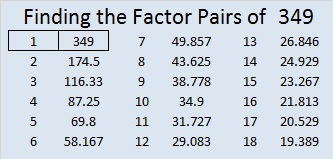# 349 and Level 6

When 2^349 is divided by 349, the remainder is 2, so 349 is VERY LIKELY a prime number. Scroll down past the puzzle to know for sure.Print the puzzles or type the factors on this excel file: 10 Factors 2015-01-05

• 349 is a prime number.
• Prime factorization: 349 is prime.
• The exponent of prime number 349 is 1. Adding 1 to that exponent we get (1 + 1) = 2. Therefore 349 has exactly 2 factors.
• Factors of 349: 1, 349
• Factor pairs: 349 = 1 x 349
• 349 has no square factors that allow its square root to be simplified. √349 ≈ 18.682

How do we know that 349 is a prime number? If 349 were not a prime number, then it would be divisible by at least one prime number less than or equal to √349 ≈ 18.682. Since 349 cannot be divided evenly by 2, 3, 5, 7, 11, 13, or 17, we know that 349 is a prime number.Prime Number Trivia: There are sixteen prime numbers between 300 and 400. Of those, 349 is the only one that doesn’t produce another prime number when 300 is subtracted from it. 349 – 300 = 49, a composite number. This piece of trivia might be helpful if you wanted to memorize all the prime numbers less than 1000.